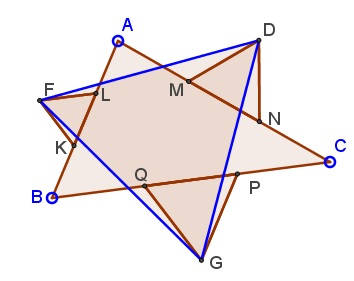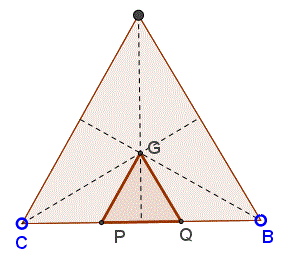### Lean Napoleon's Triangles: What is this about? A Mathematical Droodle

ExplanationThe applet attempts to suggest the following statement [Garfunkel, Stahl]:

On the middle thirds of ΔABC construct (similarly oriented) equilateral triangle, KLF, MND, and PQG. Then ΔGDF is equilateral.### Proof

The proof is said to be computational but not tedious.

ΔAKF is isosceles, with ∠AKF = 120°. So its base angles (KAF and AFK) are both 30°. It follows that ∠AFL = 90° which makes ΔAFL right. If, as usual, AB = c, then in ΔAFL, AL = 2c/3 and LF = c/3. By the Pythagorean theorem, (AF)² = c²/3.

Similarly, ΔAMD is right and (AD)² = b²/3.

We are now in a position to apply the Law of Cosines in ΔADF to determine the length of DF. Note that ∠DAF = ∠A + 60°.

 (DF)² = b²/3 + c²/3 - 2bc/3·cos(A + 60°) = b²/3 + c²/3 - 2bc/3·cos(A)cos(60°) + 2bc/3·sin(A)sin(60°) = b²/3 + c²/3 - 2bc·cos(A)/6 + 2bc/3·sin(A)√3/2 = b²/3 + c²/3 - (b² + c² - a²)/6 + 2√3/3·S = (a² + b² + c²)/6 + 2√3/3·S,

where S is the area of ΔABC. (We again used Law of Cosines and also a formula for the area of a triangle.) The expression is symmetric in a, b, c so that (GD)² and (GF)² are bound to be equal to the same quantity, proving that indeed ΔGDF is equilateral.

Observe that the above derivation only works when triangles KLF, MND, and PQG are drawn outwardly on the sides of ΔABC. If they are drawn inwardly, then ∠DAF may equal either ∠A - 60° or 60° - ∠A. In both cases we would obtain

 (DF)² = (a² + b² + c²)/6 - 2√3/3·S,

with the same conclusion. Thus we are led to two equilateral triangles related to a base ΔABC. From the above relations it follows that the difference in areas of the two triangles is exactly that of ΔABC.As James Bond drops on an occasion in "Tomorrow Never Dies", "They'll publish anything these days."

As a matter of fact, the triangle GDF is exactly Napoleon's triangle, because points G, D, F are the centers of the equilateral triangle formed on the sides of ΔABC:### References

1. J. Garfunkel, S. Stahl, The Triangle Reinvestigated, Am Math Monthly, Vol. 72, No. 1. (Jan., 1965), pp. 12-20### Napoleon's Theorem